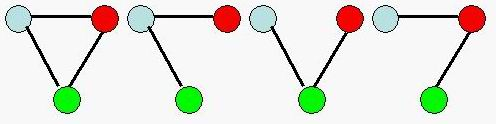Online JudgeProblem SetAuthorsOnline ContestsUser
Web Board
F.A.Qs
Statistical Charts
Problems
Submit Problem
Online Status
Prob.ID:
Register
Authors ranklist
Current Contest
Past Contests
Scheduled Contests
Award Contest
Register
Language:
Connected Graph
 Time Limit: 1000MS Memory Limit: 30000K Total Submissions: 4731 Accepted: 2114

Description

An undirected graph is a set V of vertices and a set of E∈{V*V} edges.An undirected graph is connected if and only if for every pair (u,v) of vertices,u is reachable from v.
You are to write a program that tries to calculate the number of different connected undirected graph with n vertices.
For example,there are 4 different connected undirected graphs with 3 vertices.Input

The input contains several test cases. Each test case contains an integer n, denoting the number of vertices. You may assume that 1<=n<=50. The last test case is followed by one zero.

Output

For each test case output the answer on a single line.

Sample Input

```1
2
3
4
0
```

Sample Output

```1
1
4
38
```

Source

[Submit]   [Go Back]   [Status]   [Discuss]Home PageGo BackTo top